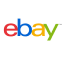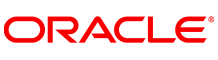New update is available. Click here to update.
Topics

# Goodness of a String

Moderate0/80
Average time to solve is 35m+1 more companies

## Problem statement

You are provided with a string ‘S’ which indicates the nested list. For example: “[1, [2, 3], [4, [5, 6] ] ]”. Each number present in the list has some depth. The depth of a particular number is the number of nested lists in which it is present. Consider the previous example in which the number ‘1’ is at depth 1, numbers ‘2’, ‘3’, and ‘4’ are at depth 2, and numbers ‘5’ and ‘6’ are at depth 3.

You have to find the goodness of the given string/nested list. The goodness of a string is the sum of the product of depths and elements present in the string.

For Example:

``````S = “[1, [2, 3], [4, [5, 6] ] ]”
Total depth  = 1*1 + 2*2 + 3*2 + 4*2 + 5*3 + 6*3 = 52
``````

Note:

``````1. The given string may be empty.
2. The string will not contain any white spaces.
3. You have to take the modulo with 10 ^ 9 + 7 as the answer may be very large.
``````
Detailed explanation ( Input/output format, Notes, Images )
Constraints:
``````1 <= T <= 100
1 <= |S| <= 100000
1 <= ES[ i ] <= 10^5

Where “|S|” is the length of the given string,  “ES[ i ]” is the element/number stored in the string at the “i-th” position.

Time limit: 1 sec
``````
Sample Input 1:
``````2
[1,[2,3],[4,[5,6]]]
[[],[]]
``````
Sample Output 1:
``````52
0
``````
Explanation of sample input 1:
``````For the first test case, the explanation is given in the description.

In the second test case, the given string hasn’t contained any element/number therefore the goodness is equal to 0.
``````
Sample Input 2:
``````3
[[[[[1,2,3,4,5]]]]]
[10,20,30,40]
[]
``````
Sample Output 2:
``````75
100
0
``````
Explanation for sample input 2:
``````In the first test case, all the numbers are at depth 5 and so the goodness is 1*5 + 2*5 + 3*5 + 4*5 + 5*5 = 75.

In the second test case, all the numbers are at depth 1 and so the goodness is 100.

In the third test case, there is no number present in the string.
``````Console